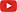## Confuse-a-Cat [General Sta­tis­tics]

Hi ElMaestro,

❝ OK, I try again.

❝ I give you two functions of a sample x, call the functions f and g (or Z and s, or alpha and beta, or apple and banana). Symbols not important. How do we determine that f and g are independent?Feel like a cat.

In the OP (and now?) you were talking about a test and whether the numerator and denominator constructing it are independent functions of $$x$$.$$t=\frac{Z}{s},\; Z=f(x)\:\wedge\:s=g(x)$$Somehow I have the feeling that the discussion moves towards transformations. Another cup of tea.

x    <- seq(1, 2, length.out = 100) fun  <- data.frame(f.1 = sin(x), f.2 = sin(x+1), f.3 = cos(x),                    f.4 = x^2,    f.5 = sqrt(x),  f.6 = tan(x)) corr <- data.frame(f.1 = rep(NA, 6), f.2 = NA, f.3 = NA,                    f.4 = NA, f.5 = NA, f.6 = NA) colnames(corr) <- rownames(corr) <- c("sin(x)", "sin(x+1)", "cos(x)",                                       "x^2", "sqrt(x)", "tan(x)") for (j in 1:nrow(corr)) {   for (k in 1:ncol(corr)) {     if (k < j) {       corr[k, j] <- sprintf("%+7.5f", cor(fun[, j], fun[, k]))     }   } } corr[is.na(corr)] <- "" corr[-nrow(corr), ]

❝ […] how about generally, when f and g are not necessarily mean and dispersion indicators of the x-sample from a normal distribution?

Are you not happy with existing tests (questioning the independence) and are trying to develop a new one? How does the “perturbation on the data” come into play?

Dif-tor heh smusma 🖖🏼 Довге життя Україна!Helmut SchützThe quality of responses received is directly proportional to the quality of the question asked. 🚮
Science QuotesIng. Helmut Schütz